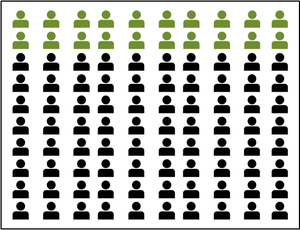# Percentage Yield Formula

• Last Updated : 06 Jan, 2022

The word “percentage” can be understood as per-cent [cent meaning 100]. So when said, (let X) is 20%, it means that if the total is 100 units then the thing (X) is 20 units. Hence if there is a total of 100 units and P items out of 100 are to be addressed then it is P%20% of people are marked green

Generally, the total count is not 100. Given a situation, there are 40 chocolates and a friend wants 50% of the total. Then how much should be given? In such cases, compute 50% of the total (here, a total of 40 chocolates are there).

FORMULA FOR CALCULATING P% OF TOTAL

Amount = (P /100) × Total

The above formula has been derived from the Unitary method,

If 100 units have P units

Then total units will have (P/100) × Total.

So continuing from the example, give (50/100) × 40 = 40/2 = 20 chocolates to the friend.

Suppose, only 15 chocolates are to be given to the friend. Then what percentage of the total share is to be given?

Required percentage = (Amount/ Total ) × 100 %

= (15/40) × 100 %

= 37.5%

OR by using the same previous formula,

Let you are giving x% to the friend then,

15 = (x/100) × 40

15 × 100/40 = x

x = 37.5%

Thus, will give 37.5% of the total chocolates.

### Percentage Yield Formula

Percentage yield can be defined as the amount of product that is actually made when compared to the maximum yield calculated is called the percentage yield. The formula for percentage yield is given as,

Percentage yield = [(Output/Actual value)/(Calculated/ Theoritical value)] × 100%

### Sample Problems

Question 1: Solve the following,

1. 35% of 700
2. 0.5% of 4
3. 78%

Solution:

1. 35% of 700 = (35/100) × 700 = 35 × 7 = 245
2. 0.5% of 4 = (0.5/100) × 4 = 2.0/100 = 0.02
3. 78% means 78/100 = 0.78

Question 2: What is a 40% discount on a product worth Rs. 599?

Solution:

Here the discount = 40% of Rs. 599 = 0.40 × 599 = Rs. 239.60

Question 3: Mr. John Doe has a monthly income of Rs. 45,273. He invests 23.5% of his total income. He saves 35% of the remaining amount. Rest are his monthly expenditure. Calculate the amount invested and saved, and the percentage of his expenditure.

Solution:

Here the total amount is Rs. 45273.
Amount for investment = 23.5% of Rs. 45273 = (23.5/100) × 45273 = Rs. 10639.16 (Rounded off to 2 decimals).
Amount saved = 35% of Remaining = (35/100) × (45273 – 10639.16) = 0.35 × 34633.845 = Rs. 12121.85.
Amount of expenditure = Total income – Invested amount – Amount saved = 45273 – 10639.16 – 12121.85 = Rs. 22511.99.
Percentage of Expenditure = Amount of expenditure/total Amount × 100% = (22511.99/45273) × 100% = 49.72%

Question 4: While doing a chemical lab experiment, the product obtained weighed 0.45g. Before the experiment, the weight was calculated to be 11g. Calculate the percentage yield.

Solution:

Percentage yield = (Actual/Theoretical) × 100 = (0.45/11) × 100% = 4.09%.

Question 5: While doing a chemical lab experiment, the product obtained weighed 8g. Before the experiment, the weight was calculated to be Xg. The percentage yield is 60%. Find X.

Solution:

Percentage yield = (Actual/Theoretical) × 100%.

60% = (8/X) × 100%

X = 8 × 100/60 = 13.33.

Question 6: While doing a chemical lab experiment, the product obtained weighed Xg. Before the experiment, the weight was calculated to be 4g. The percentage yield is 34.5%. Find X.

Solution:

Percentage yield = (Actual/Theoretical) × 100

34.5% = (X/4) × 100%

X = 34.5 × 4/100 = 1.38

Question 7: In experiment-1, the weight of the output product is calculated to be 14g. The percentage yield was calculated to be 48%. The output obtained is 30% of the weight of another product in experiment-2 whose weight was calculated to be 56g. Find the percentage yield of experiment-2.

Solution:

In Experiment-1, Let output obtained = Xg

48% = (X/14) × 100%

X = (48 × 14)/100 = 6.72

Now, X is 30% of  Y, where Y = Output of Experiment-2,

X = (30/100) × Y

6.72 × 100/30 = Y = 22.4g.

Thus Percentage Yield of experiment-2 = (22.4/56) × 100 = 40%

NOTE:  While calculating percentage, multiply by 100; while using a given percentage, divide by 100.

My Personal Notes arrow_drop_up
Recommended Articles
Page :